# Methods and formulas for Laney P' Chart

Select the method or formula of your choice.

## Plotted points

Each plotted point represents the proportion of defectives for one subgroup, pi:

Data points on a P chart follow the binomial distribution.

### Notation

TermDescription
xinumber of defectives for subgroup i
nisize of subgroup i

## Center line

The center line represents the proportion of defectives for your process,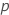. If you do not specify a historical value, then Minitab uses the average proportion of defectives from your data,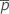, to estimate.

### Notation

TermDescription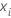number of defectives in subgroup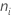size of subgroup## Sigma Z

Each subgroup proportion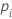is converted to a z-score (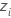):

Next, a moving range of length 2 is used to evaluate the variation in the z-scores and calculate Sigma Z (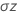):

where 1.128 is an unbiasing constant.

### Notation

TermDescriptionproportion of defectives for subgroupprocess proportionsize of subgroup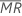moving range of length 2

## Control limits

### Lower control limit (LCL)

The LCL for each subgroupis equal to the greater of the following:

or

### Upper control limit (UCL)

The UCL for each subgroupis equal to the lesser of the following:

or

### Notation

TermDescriptionprocess proportion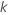parameter for Test 1. The default is 3. (For more information, go to Select tests for special causes for Laney P' Chart.)size of subgroupsee "Sigma Z"
By using this site you agree to the use of cookies for analytics and personalized content.  Read our policy